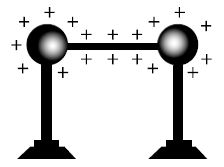Physics

# Explain Measurement of Electric Potential

The work done to bring a unit positive charge from infinity to a point in an electric field is called the potential of that point. Again from infinity if a unit of positive charge is brought near to the conductor, the work done by the electric force or against the electric force is called potential of that conductor. If a unit positive charge q is brought very near to the conductor from a point at infinity and if the amount of work done is W, the potential ‘V’ of the conductor or of that point will be, V = W/q

Electric potential determines in which direction the flow of electric charge takes place when two charged conductors are electrically connected. If two positively charged metallic spheres are connected by conducting wire (fig) then any of the following phenomena may occur.1. Some charge from the left sphere may go to the right sphere.
2. Some charge from the right sphere may go to the left sphere
3. The charges may remain as it is.

The movement of charge from one sphere to another does not depend on the quantity of charge of the spheres but it depends on electric potential. The positive charge will flow from sphere to sphere of higher potential to that if lower potential. This flow of charge will continue until the potential of these two spheres become equal. So, potential is an electric condition of a charged conductor that determines whether it takes or gives up charge when connected to another charged conductor by a connecting wire.